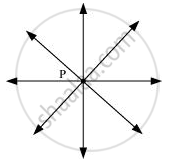# Only one line can pass through a single point. - Mathematics

MCQ
True or False

Only one line can pass through a single point.

• True

• False

#### Solution

False.

Reason - Since through a single point, infinite number of lines can pass. In the following figure, it can be seen that there are infinite numbers of lines passing through a single point P.Concept: Euclid’S Definitions, Axioms and Postulates
Is there an error in this question or solution?
Chapter 5: Introduction to Euclid's Geometry - Exercise 5.1 [Page 85]

#### APPEARS IN

NCERT Class 9 Maths
Chapter 5 Introduction to Euclid's Geometry
Exercise 5.1 | Q 1. (i) | Page 85

Share